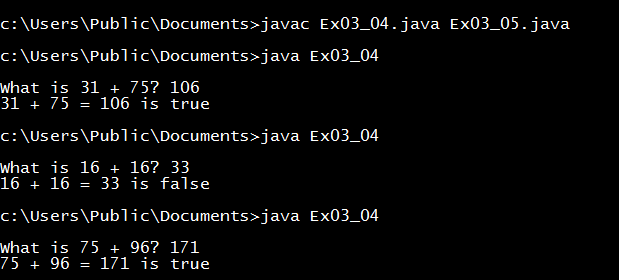# Exercise 3.4: Game: Learn Addition

Program that generates two integers under 100 and prompts the user to enter the sum of these two integers.
```/**
*
* @Author: Aghatise Osazuwa
* Website: www.cscprogrammingtutorials.com
*/

import java.util.Scanner;

public class Ex03_04 {
public static void main(String[] args) {
int number1 = (int)(System.currentTimeMillis() % 100);
int number2 = (int)(System.currentTimeMillis() / 7 % 100);
Scanner input = new Scanner(System.in);
System.out.print("What is " + number1 + " + " + number2 + "? ");
int answer = input.nextInt();
System.out.println(number1 + " + " + number2 + " = " + answer + " is "
+ (number1 + number2 == answer));
}
}
```Sample run

Click here to see other solutions to Introduction to Java Programming.